Next: An estimation of the Up: The Trust-Region subproblem Previous: The Rayleigh quotient trick   Contents

Subsections

# Termination Test.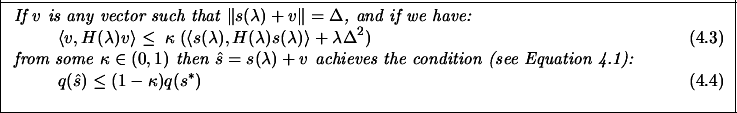In other words, if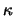is small, then the reduction in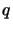that occurs at the pointis close to the greatest reduction that is allowed by the trust region constraint.
Proof
for any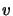, we have the identity: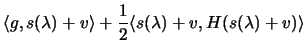( using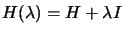: )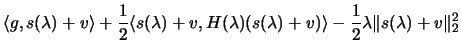( using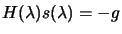: )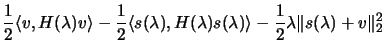(4.23)

If we choosesuch that, we have: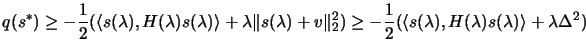(4.24)

From 4.27, using the 2 hypothesis:( Using Equation 4.25: )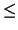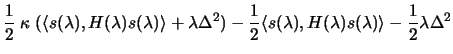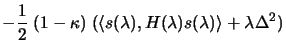(4.25)

Combining 4.28 and 4.29, we obtain finally 4.26.

##is near the boundary of the trust region: normal case

Lemma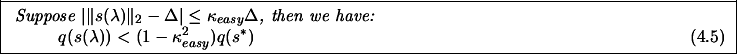From the hypothesis: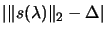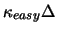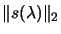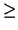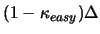(4.26)

Combining 4.31 and 4.27 when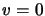reveals that: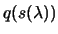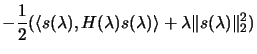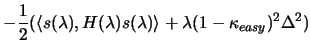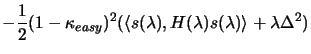(4.27)

The required inequality 4.30 is immediate from 4.28 and 4.32.
We will use this lemma with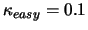.

##is inside the trust region: hard case

We will chooseas (see paragraph containing Equation 4.15 for the meaning of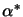and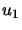):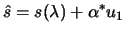(4.28)

Thus, the condition for ending the trust region calculation simplifies to the inequality: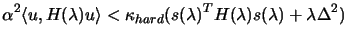(4.29)

We will choose.Next: An estimation of the Up: The Trust-Region subproblem Previous: The Rayleigh quotient trick   Contents
Frank Vanden Berghen 2004-04-19